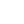# Definition of Dynamic Programming

Copy from wikipedia

dynamic programming is a method for solving a complex problem by breaking it down into a collection of simpler subproblems, solving each of those subproblems just once, and storing their solutions - ideally, using a memory-based data structure. The next time the same subproblem occurs, instead of recomputing its solution, one simply looks up the previously computed solution, thereby saving computation time at the expense of a (hopefully) modest expenditure in storage space. (Each of the subproblem solutions is indexed in some way, typically based on the values of its input parameters, so as to facilitate its lookup.) The act of storing solutions to subproblems is called "memoization".

• one of the divide and conquer method and use a table for memorialization of temporary(smaller problem) result.
• executive, considering every possibility.
• usually done with bottom up approach in implementation (start from 0 of the array)
• quite independent in each subproblem, each result of the subproblem is a good, complete solution of that subproblem.
• usually in structure of OPT(subproblem) + xxxx
• Usually YES _ NO situation? INCLUDENOTINCLUDE situation?

# Weighted Interval Scheduling Problem

Consider whether a job j is chosen to be included or not

1. If include consider it last compatible job (p(j) + Vj
2. If not consider it previous job
```Sort by the finish time
OPT(j) = 0 if j = 0
OPT(j) = max(OPT(j - 1), OPT(p(j) + Vj)
```

Compute p(n)

```sort one list with start time (1)
sort one list with finish time (2)
loop from the end until (1).start > finish
```

# Longest Common Subsequence

X, Y be two sequence of letters, Find their longest common subsequence Consider whether a letter X[i], Y[j] equal or not

1. Either one of them is empty, zero in common
2. They are the same, the LCS of without both letter + 1
3. They are different, max LCS of missing either one of the letter
```  LCS[i, j] = 0 if i or y = 0
LCS[i, j] = LCS[i - 1, j - 1] + 1, X[i] == Y[j]
LCS[i, j] = max(LCS[i-1, j], LCS[i, j-1]), X[i] != Y[j]
```

# Shortest common superseuqnece

X, Y be two sequence of letters, Find super subersequence of them (superset of both characters) Consider whether a letter X[i], Y[j] equal or not

1. Either one of them is empty, |j| or |i|
2. Not equal, try to include X or try to include Y in the supersequence, compare SCS from missing either one of the letters + 1
3. Equal, SCS from missing both letters + 1

```SCS = |j| if i is 0
SCS = |i| if j is 0
SCS = min(SCS[i-1, j] + 1, SCS[i, j-1] + 1)
SCS = SCS[i-1, j-1] + 1
```

# 0-1 Knapsack Problem

Consider a set of items. consider include item i or not

1. no items include yet, of course zero value
2. cannot include the item due to exceed of weight limit
3. not include the item, consider OPT from previous items VS include the item, consider OPT from the (bag weight - item weight) + item value
```OPT[i, W] = 0 if i = 0
OPT[i, W] = OPT[i - 1, W] Wi >= W
OPT[i, W] = max(OPT[i - 1, W], OPT[i - 1 , W - Wi] + Vi) if W >= Wi
```